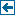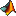Master index Index for chronux/spectral_analysis/helper# Index for chronux/spectral_analysis/helper

## Matlab files in this directory:change_row_to_column Helper routine to transform 1d arrays into column vectors that are neededcheck_consistency Helper routine to check consistency of data dimensionscoherr Function to compute lower and upper confidence intervals on the coherencycohmathelper Helper function called by coherency matrix computations.den_jack Function to compute smooth estimates of the mean of x using locfit,dpsschk Helper function to calculate tapers and, if precalculated tapers are supplied,getfgrid Helper function that gets the frequency grid associated with a given fft based computationgetparams Helper function to convert structure params to variables used by thejackknife Compute jackknife estimates of the mean and standard deviation of input data xspecerr Function to compute lower and upper confidence intervals on the spectrum

Generated on Fri 28-Sep-2012 12:34:27 by m2html © 2005